## Classification

#### Classification

Direction: In each problem, out of the five figures marked (a), (b), (c), (d) and (e), four are similar in a certain manner. However one figure is not like the other four. Choose the figure which is different from the rest.

1. Choose the figure which is different from the rest.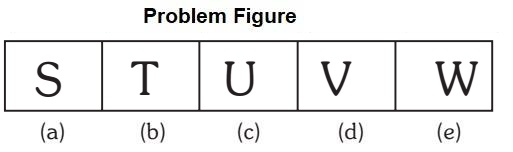1. Only one letter is vowel.

##### Correct Option: C

Only U is vowel,rest are consonant.

1. Choose the figure which is different from the rest.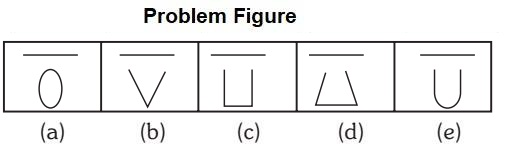1. One element is enclosed figure.

##### Correct Option: A

One element is enclosed figure, rest are one end open.

1. Choose the figure which is different from the rest.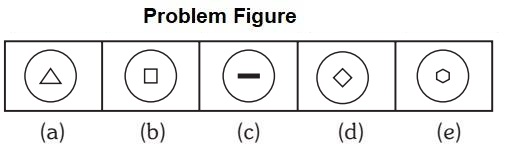1. All figure has enclosed figure except one.

##### Correct Option: C

All except (c) has enclosed figure.

1. Choose the figure which is different from the rest.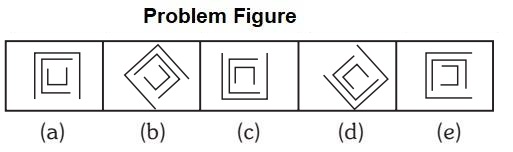1. All other figure can be rotated into each other.

##### Correct Option: A

All other figure can be rotated into each other. The middle element is obtained by rotating the outer element through 90 degree clock wise and the inner element is obtained by rotating the middle element through 90 degree clock wise.

1. Choose the figure which is different from the rest.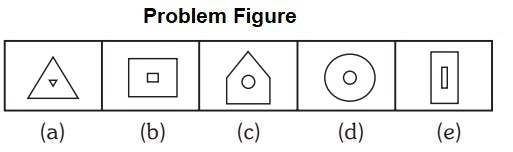1. Elements having the replica of its present inside of that.

##### Correct Option: C

All outer elements having the replica of its present inside of that, except option (c)